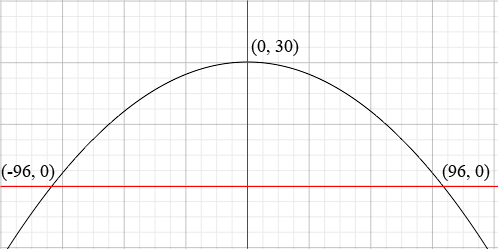SEARCH HOMEMath Central Quandaries & QueriesQuestion from megan, a student: A bridge is built in the shape of a parabolic arch. The bridge has a span of 192 feet and a maximum height of 30 feet. Find the height of the arch at 20 feet from its center. I need the equation and what to fill into the equation...please and thankyou!Hi Megan,

Draw a coordinate system on the plane with the x-axis at ground level and the y-axis the axis of symmetry of the parabola. The width of the bridge is 192 feet so the parabola crosses the x-axis with x-coordinates ± 192/2 = ± 96. The maximum height occurs at x = 0 so the vertex of the parabola is (0, 30).Since the curve is a parabola which opens downward its equation can be written f(x) = ax2 + bx + c.

Since (0, 30) is on the curve f(0) = 30. What does that tell you about c?
f(96) = f(-96). What does that tell you about b?
f(96) = 0. What does that tell you about a?

I hope this helps,
PennyMath Central is supported by the University of Regina and The Pacific Institute for the Mathematical Sciences.